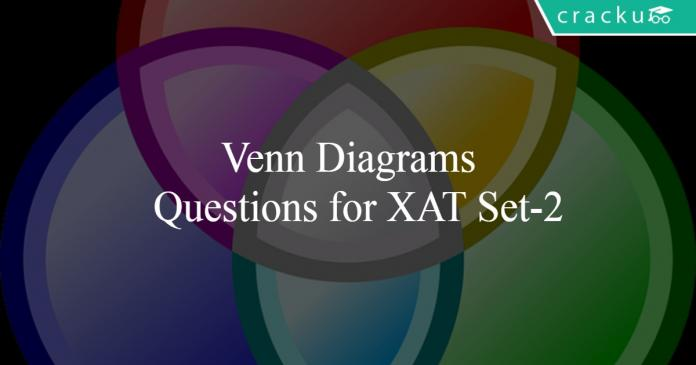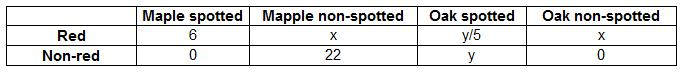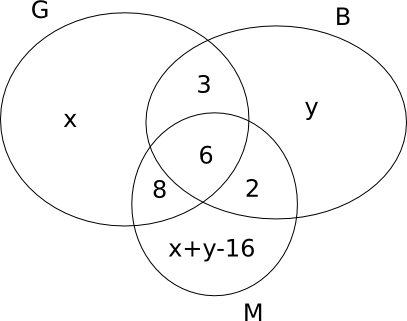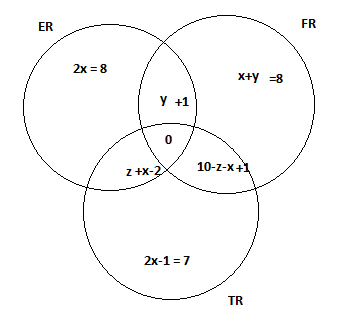# Venn Diagrams Questions for XAT Set-2

0
333Venn Diagrams Questions for XAT Set-2

# Venn Diagrams Questions for XAT Set-2

Download important Venn Diagram Questions Set-2 for XAT  Set-2 PDF based on previously asked questions in the XAT exam. Practice Venn Diagram Questions  Set-2 PDF for the XAT exam.

Question 1: 70% of the students who joined XCRI last year play football, 75% play cricket, 80% play basketball and 85% play carrom. The minimum percentage of students who play all four games is:

a) 5%

b) 10%

c) 15%

d) 20%

e) None of the above

Question 2: For two sets A and B, let AΔB denote the set of elements which belong to A or B but not both. If P = {1,2,3,4}, Q = {2,3,5,6,}, R = {1,3,7,8,9}, S = {2,4,9,10}, then the number of elements in (PΔQ)Δ(RΔS) is

Question 3: A club has 256 members of whom 144 can play football, 123 can play tennis, and 132 can play cricket. Moreover, 58 members can play both football and tennis, 25 can play both cricket and tennis, while 63 can play both football and cricket. If every member can play at least one game, then the number of members who can play only tennis is

a) 38

b) 32

c) 45

d) 43

Question 4: There are 240 second year students in a B – School. The Finance area offers 3 electives in the second year. These are Financial Derivatives, Behavioural Finance, and Security Analysis. Four students have taken all the three electives, and 48 students have taken Financial Derivatives. There are twice as many students who study Financial Derivatives and Security Analysis but not Behavioural Finance, as those who study both Financial Derivatives and Behavioural Finance but not Security Analysis, and 4 times as many who study all the three. 124 students study Security Analysis. There are 59 students who could not muster courage to take up any of these subjects. The group of students who study both Financial Derivatives and Security Analysis but not Behavioural Finance, is exactly the same as the group made up of students who study both Behavioural Finance and Security Analysis. How many students study Behavioural Finance only?

a) 29

b) 30

c) 32

d) 35

e) None of the above

Question 5: There are 3 clubs A, B & C in a town with 40, 50 & 60 members respectively. While 10 people are members of all 3 clubs, 70 are members in only one club. How many belong to exactly two clubs?

a) 20

b) 25

c) 50

d) 70

Question 6: On her walk through the park, Hamsa collected 50 coloured leaves, all either maple or oak. She sorted them by category when she got home, and found the following:The number of red oak leaves with spots is even and positive.The number of red oak leaves without any spot equals the number of red maple leaves without spots.All non-red oak leaves have spots, and there are five times as many of them as there are red spotted oak leaves.There are no spotted maple leaves that are not red.There are exactly 6 red spotted maple leaves.There are exactly 22 maple leaves that are neither spotted nor red.How many oak leaves did she collect?

a) 22

b) 17

c) 25

d) 18

Instructions

DIRECTIONS for the following two questions: Answer the questions on the basis of the information given below.

New Age Consultants have three consultants Gyani, Medha and Buddhi. The sum of the number of projects handled by Gyani and Buddhi individually is equal to the number of projects in which Medha is involved. All three consultants are involved together in 6 projects. Gyani works with Medha in 14 projects. Buddhi has 2 projects with Medha but without Gyani, and 3 projects with Gyani but without Medha. The total number of projects for New Age Consultants is one less than twice the number of projects in which more than one consultant is involved.

Question 7: What is the number of projects in which Medha alone is involved?

a) Uniquely equal to zero.

b) Uniquely equal to 1.

c) Uniquely equal to 4.

d) Cannot be determined uniquely.

Instructions

Help Distress (HD) is an NGO involved in providing assistance to people suffering from natural disasters. Currently, it has 37 volunteers. They are involved in three projects: Tsunami Relief (TR) in Tamil Nadu, Flood Relief (FR) in Maharashtra, and Earthquake Relief (ER) in Gujarat. Each volunteer working with Help Distress has to be involved in at least one relief work project.

• A Maximum number of volunteers are involved in the FR project. Among them, the number of volunteers involved in FR project alone is equal to the volunteers having additional involvement in the ER project.
• The number of volunteers involved in the ER project alone is double the number of volunteers involved in all the three projects.
• 17 volunteers are involved in the TR project.
• The number of volunteers involved in the TR project alone is one less than the number ofvolunteers involved in ER project alone.
• Ten volunteers involved in the TR project are also involved in at least one more project.

Question 8: After some time, the volunteers who were involved in all the three projects were asked to withdraw from one project. As a result, one of the volunteers opted out of the TR project, and one opted out of the ER project, while the remaining ones involved in all the three projects opted out of the FR project. Which of the following statements, then, necessarily follows?

a) The lowest number of volunteers is now in TR project.

b) More volunteers are now in FR project as compared to ER project.

c) More volunteers are now in TR project as compared to ER project.

d) None of the above.

Question 9: After the withdrawal of volunteers, as indicated in the previous question, some new volunteers joined the NGO. Each one of them was allotted only one project in a manner such that, the number of volunteers working in one project alone for each of the three projects became identical. At that point, it was also found that the number of volunteers involved in FR and ER projects was the same as the number of volunteers involved in TR and ER projects. Which of the projects now has the highest number of volunteers?

a) ER

b) FR

c) TR

d) Cannot be determined

Question 10: Fifty college teachers are surveyed as to their possession of colour TV, VCR and tape recorder. Of them, 22 own colour TV, 15 own VCR and 14 own tape recorders. Nine of these college teachers own exactly two items out of colour TV, VCR and tape recorder; and, one college teacher owns all three. How many of the 50 teachers own none of the three, colour TV, VCR or tape recorder?

a) 4

b) 9

c) 10

d) 11

Answers & Solutions:

1) Answer (B)

Let ‘100x’ be the number of students who joined XCRI last year.

Let ‘a’, ‘b’, ‘c’ and d be the number of students who play 1 game, 2 games, 3 games and 4 games respectively.

Therefore,

a+b+c+d = 100x … (1)

a+2b+3c+4d = 70x+75x+80x+85x

a+2b+3c+4d = 310x … (2)

By equation (2) – (1)

b+2c+3d = 210x

We have to minimize ‘d’ for that we have to maximize c. But c $\leq$ 100x

At c$_{max}$ = 90x, d$_{min}$ = 10x

Therefore, we can say that the minimum percentage of students who play all four games = 10%.

2) Answer: 7

P = {1,2,3,4} and  Q = {2,3,5,6,}
PΔQ = {1, 4, 5, 6}
R = {1,3,7,8,9} and S = {2,4,9,10}
RΔS = {1, 2, 3, 4, 7, 8, 10}
(PΔQ)Δ(RΔS) = {2, 3, 5, 6, 7, 8, 10}
Thus, there are 7 elements in (PΔQ)Δ(RΔS) .
hence, 7 is the correct answer.

3) Answer (D)

Assume the number of members who can play exactly 1 game = I

The number of members who can play exactly 1 game = II

The number of members who can play exactly 1 game = III

I+2II+3III=144+123+132=399….(1)

I+II+III=256……(2)

=> II+2III=143…..(3)

Also, II+3III=58+25+63=146 ……(4)

=> III = 3 (From 3 and 4)

=> II =137

=> I = 116

The members who play only tennis = 123-58-25+3 = 43

4) Answer (A)

Given : $e = 4$

$FD = 48$, => $a + b + d + e = 48$

$d = 2b$ and $d = 4e$

$SA = 124$, => $d + e + f + g = 124$

$d = e + f$ and $h = 59$

To find : $c = ?$

Solution : $d = 4e = 4 \times 4 = 16$

=> $b = \frac{d}{2} = \frac{16}{2} = 8$

=> $f = d – e = 16 – 4 = 12$

=> $a = 48 – b – d – e = 48 – 8 – 16 – 4 = 20$

=> $g = 124 – d – e – f = 124 – 16 – 4 – 12 = 92$

Now, we know that, $a + b + c + d + e + f + g + h = 240$

=> $20 + 8 + c + 16 + 4 + 12 + 92 + 59 = 240$

=> $c + 211 = 240$

=> $c = 240 – 211 = 29$

5) Answer (B)

We know that x + y + z = T and x + 2y + 3z = R, where
x = number of members belonging to exactly 1 set = 70
y = number of members belonging to exactly 2 sets
z = number of members belonging to exactly 3 sets = 10
T = Total number of members
R = Repeated total of all the members = (40+50+60) = 150
Thus we have two equations and two unknowns. Solving this we get y = 25

So, 25 people belong to exactly 2 clubs.

6) Answer (B)6+x+22+x+6y/5 = 50
=> 5x + 3y = 55
Since y/5 is even, y should be a multiple of 10
The only possible value is 10
So, y = 10 and x = 5
No. of oak leaves = 17

7) Answer (B)The total number of projects = 2(3+6+8+2) – 1 = 38 – 1 = 37
So, 19 + 2(x+y) – 16 = 37
=> x+y = 17
The number of projects in which Medha alone is involved is 17-16 = 1
But the number of projects in which Gyani alone is involved cannot be uniquely determined

8) Answer (B)So we can see that even if volunteers are withdrawn , the number of volunteers in FR are more as in, total if we calculate comes out to be more. Hence option B.

9) Answer (A)

Consider p volunteers be added to TR project and q be added to each of FR and ER projects.

Then, 7 + p = 8 + q => p = q + 1

Also, Number of volunteers working on TR = 7 + q + 1 + 4 + 5 = 17 + q

Number of volunteers working on FR = 17 + q

Number of volunteers working on ER = 18 + q.

So if we take any values we get ER  greater than both FR and TR.

10) Answer (C)

Number of people owning exactly 2 articles = 9

Number of people owning exactly 3 articles = 1

Applying AUBUC formula, we get

AUBUC = 22+15+14 – 9 -2*(1)=40

Number of people who do not own any article = 50-40 = 10

We hope this Venn Diagram Questions Set-2 PDF for XAT with Solutions will be helpful to you.